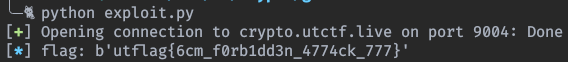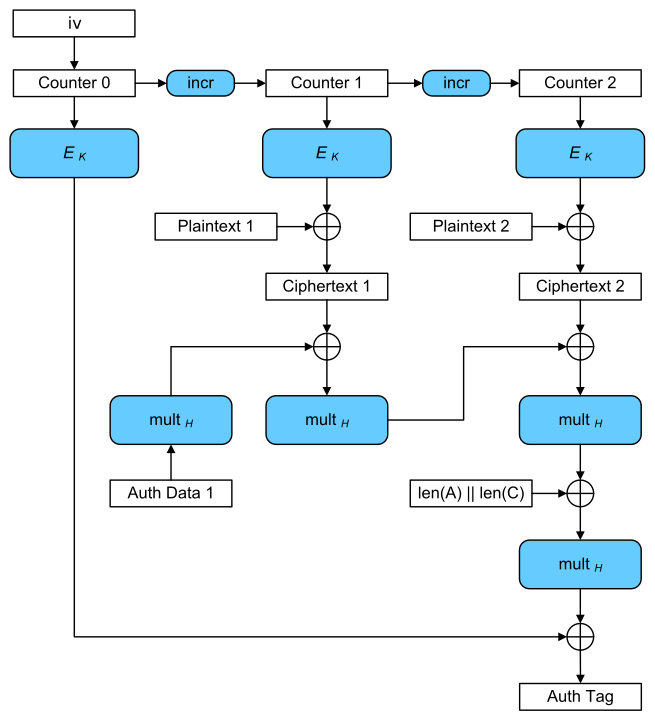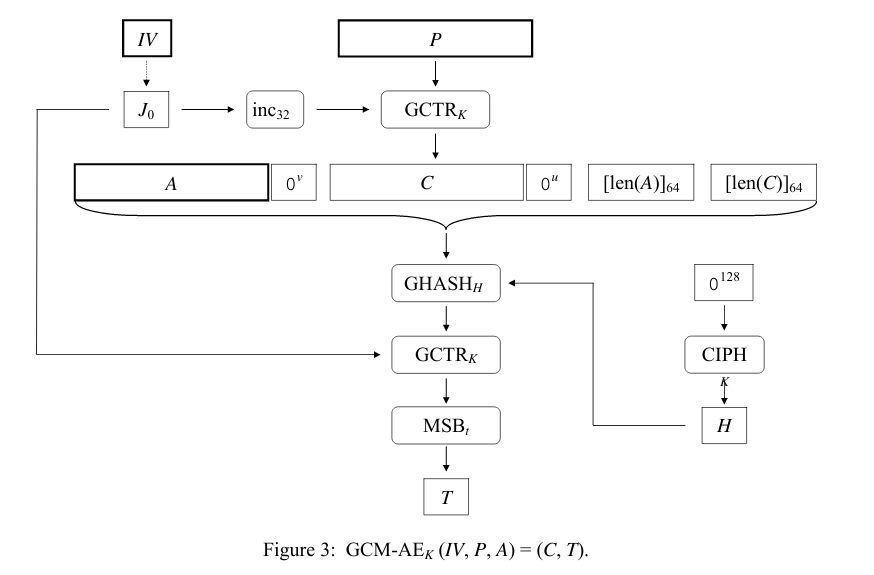Galois, Curveball.

# Galois

Attack nonce reuse on AES-GCM with CTR two time pad and forbidden attack.

## Information

• category : crypto
• points : 1072

## Description

nc crypto.utctf.live 9004

1 file: server.py

## Writeup

Let’s look server.py:

#!/usr/bin/env python3

import sys
from Crypto.Cipher import AES
from Crypto.Random import get_random_bytes
from secret import flag

KEY = get_random_bytes(16)
NONCE = get_random_bytes(16)

def aes_gcm_encrypt(plaintext):
cipher = AES.new(KEY, AES.MODE_GCM, nonce=NONCE)
ciphertext, tag = cipher.encrypt_and_digest(plaintext)
return ciphertext.hex(), tag.hex()

def aes_gcm_decrypt(ciphertext, tag):
cipher = AES.new(KEY, AES.MODE_GCM, nonce=NONCE)
plaintext = cipher.decrypt_and_verify(ciphertext, tag)
return plaintext

if __name__ == '__main__':
options = '''Welcome to the AES GCM encryption and decryption tool!
1. Encrypt message
2. Decrypt message
3. Quit
'''

def encrypt_msg():
print("Input a string to encrypt (must be at least 32 characters):")
user_input = input()
if len(user_input) < 32:
sys.exit()
output = aes_gcm_encrypt(user_input.encode())
print("Here is your encrypted string & tag, have a nice day :)")
print(output)

def decrypt_msg():
print("Input a hex string and its tag to decrypt:")
user_input = bytearray.fromhex(input())
tag = bytearray.fromhex(input())
try:
output = aes_gcm_decrypt(user_input, tag)
except ValueError:
print("Decryption failed :(")
return
print("Here is your decrypted string, have a nice day :)")
print(output)

def quit():
sys.exit()

'1' : encrypt_msg,
'2' : decrypt_msg,
'3' : quit
}

i = 0
print('flag', aes_gcm_encrypt(flag))
while i < 10:
print(options)
print('Select option: ')
choice = input()
print("Not a valid choice...")
sys.exit()
i += 1


The server uses AES-GCM as encryption scheme and permits the following operations:

1. Encrypt msg: to encrypt every message we want.
• Input: msg, with len(msg) >= 32.
• Output: pair (ciphertext, tag).
2. Decrypt ciphertext: to decrypt a ciphertext (lol).
• Input: pair(ciphertext, tag).
• Output: ciphertext decrypted.

The server prints also the encryption of the flag (without tag). Our scope is to decrypt the flag.

AES-GCM is an AEAD encryption scheme, which means that unlike AES-CBC or AES-CTR it also generates a tag (MAC) to authenticate the message. To do that it uses some operations over $$GF(2^{256}$$), which are really cool (explained later).

Looking at the source code of the server it’s easy to see that the nonce is reused. The encryption part of the plaintext is equal to the one used in CTR mode. A security risk of CTR mode is to never reuse the IV (Nonce || counter) twice (or more).

Why?

Well, we have a two-time-pad attack, since the plaintext is xored with the same keystream twice. The attack is the following:

flag_enc = xxxx # Take the encrypted flag from the server
ct, tag = AES_GCM(k, payload) # query the server
flag = ct xor payload xor flag_enc =
e(k, iv) xor payload xor payload xor e(k, iv) xor flag =
flag


## Exploit

#!/usr/bin/env python3

from pwn import remote, log

def xor(s1, s2):
if(len(s1) == 1 and len(s1) == 1):
return bytes([ord(s1) ^ ord(s2)])
else:
return bytes(x ^ y for x, y in zip(s1, s2))

def main():
conn = remote('crypto.utctf.live', 9004)
conn.recv(5)
flag_enc = bytes.fromhex(conn.recv(64).decode())
conn.recv()
conn.sendline('1')
conn.recvline()
conn.recvline()
ciphertext = bytes.fromhex(conn.recv(66).decode()[2:])
# flag_enc xor ciphertext xor payload =
# e(k, iv) xor flag xor e(k, iv) xor payload xor payload =
# flag
log.info("flag: " + str(flag))

if __name__ == '__main__':
main()


Launch the exploit:## Forbidden Attack

The two time pad is not the only attack on AES-GCM, since a nonce reuse opens the door to the forbidden attack, which is used to generate a valid tag. Let’s explore better how AES-GCM works:This is the wikipedia scheme, however is not completely correct since the counter starts at $$1$$, and the ciphertext and associated data is not padded in the figure.

The next scheme is the NIST one, which is more correct (but harder to understand maybe)The IV is composed by a 96 bit Nonce + 32 bit counter. We need to define the following variables:

• $$K =$$ AES key.
• $$P =$$ plaintext.
• $$C =$$ ciphertext.
• $$A =$$ associated data.
• $$H = AES(K, 0^{128})$$.
• $$J_0 = Nonce || 0^{31}1$$.
• $$EJ = AES(K, J_0)$$.
• $$GHASH_H(X) = H * X \in GF(2^{128})$$ with irreducible polynomial $$x^{128} + x^7 + x^2 + x + 1$$.
• $$L = L_a + L_c$$.

Now, why in one figure we have a xor, while in the other not?

Simply because addition in $$GF(2^{128})$$ is equal to the xor operation.

Let’s explain better how the $$GHASH_H$$ part works: If we call $$AES-GCM(K, C, A)$$ where:

• $$K$$ = Key
• $$C$$ = Ciphertext (ex. long 32 bytes = 2 blocks of 128 bit)
• $$A$$ = Associated data (ex. long 14 bytes = 1 block padded to 128 bit).

To generate the tag $$T$$ the algorithms will do:

$$T = (((((((H * A) + C_1) * H) + C_2) * H) + L) * H) + EJ$$

which is equal to:

$$T = A * H^4 + C_1 * H^3 + C_2 * H^2 + L * H + EJ$$

So we construct the polynomial:

$$G(X) = A * X^4 + C_1 * X^3 + C_2 * X^2 + L * H + EJ$$

and we compute the tag as: $$T = G(H)$$.

As an attacker we know everything except $$H$$ and $$EJ$$.

What happens when two message are encrypted/authenticated using the same nonce?

Well, let’s call $$G_1(X)$$ the polynomial on the first message, and $$G_2(X)$$ the polynomial of the second message and the messages have the same length.

• $$G_1(X) = C_{1,1} * X^3 + C_{1,2} * X^2 + L * H + EJ$$
• $$G_2(X) = C_{2,1} * X^3 + C_{2,2} * X^2 + L * H + EJ$$

Now we don’t know $$EJ$$, so it’s impossible for us to compute the tag, however if we sum the polynomial together we have:

$$P(X) = (C_{1,1} + C_{2,1}) * X^3 + (C_{1,2} + C_{2,2}) * X^2 + (L + L) * H + (EJ + EJ)$$

$$P(X) = (C_{1,1} + C_{2,1}) * X^3 + (C_{1,2} + C_{2,2}) * X^2$$

Now if we add to $$P(X)$$ the two tags, and sobstitute to $$X, H$$:

$$T_1 + T_2 = (C_{1,1} + C_{2,1}) * H^3 + (C_{1,2} + C_{2,2}) * H^2$$

Move the tags to the other side (in $$GF(2^{m})$$ addition and substraction are equal).

$$0 = (C_{1,1} + C_{2,1}) * H^3 + (C_{1,2} + C_{2,2}) * H^2 + (T_1 + T_2)$$.

Now what we have to do is to simply get the root of this equation, which will have at most $$3$$ solutions (degree of the polynomial).

Once we found $$H$$, to find the missing value $$EJ$$, we just have to compute:

$$EJ = C_{1,1} * H^3 + C_{1,2} * H^2 + L * H + T1$$.

Now we can compute the authentication tag for every ciphertext. In this case we can find the tag for the encrypted flag, and asks the server to decrypted it. In real world this is very dangerous, since the encryption of the ciphertext is simply a xor operation we can manipulate easily the decrypted plaintext (if we have the capability of a KPA).

## Exploit

Sage exploit:

#!/usr/bin/env sage

from sage.all import *   # import sage library
from Crypto.Util.number import long_to_bytes as lb
from Crypto.Util.number import bytes_to_long as bl
from binascii import unhexlify, hexlify
from sage.all import *
import struct

def bytes_to_polynomial(block, a):
poly = 0
bin_block = bin(bl(block))[2 :].zfill(128)
# reverse it to count correctly, wrong don't reverse it lol
# bin_block = bin_block[::-1]
for i in range(len(bin_block)):
poly += a^i * int(bin_block[i])
return poly

def polynomial_to_bytes(poly):
return lb(int(bin(poly.integer_representation())[2:].zfill(128)[::-1], 2))

def convert_to_blocks(ciphertext):
return [ciphertext[i:i + 16] for i in range(0 , len(ciphertext), 16)]

def xor(s1, s2):
if(len(s1) == 1 and len(s1) == 1):
return bytes([ord(s1) ^^ ord(s2)])
else:
return bytes(x ^^ y for x, y in zip(s1, s2))

F, a = GF(2^128, name="a", modulus=x^128 + x^7 + x^2 + x + 1).objgen()
R, x = PolynomialRing(F, name="x").objgen()

# Set correct values
C1 = convert_to_blocks(bytes.fromhex("8f11899ff254a177e3fb96a3d9fbf3bd7988a3e4dd693ba2515cc6a9d44f830a"))
T1 = bytes.fromhex("ac88b484d0258ca4094f459b920d46c7")
C3 = convert_to_blocks(bytes.fromhex("9b048e92f252bb20e1f7a8a488e8f0ed7c8df1ebe33c6df4045ecc978219d516"))

L = struct.pack(">QQ", 0 * 8, len(C1) * 8)
C1_p = [bytes_to_polynomial(C1[i], a) for i in range(len(C1))]
C2_p = [bytes_to_polynomial(C2[i], a) for i in range(len(C2))]
C3_p = [bytes_to_polynomial(C3[i], a) for i in range(len(C3))]
T1_p = bytes_to_polynomial(T1, a)
T2_p = bytes_to_polynomial(T2, a)
L_p = bytes_to_polynomial(L, a)
# Here G_1 is already modified to include the tag
G_1 = (C1_p * x^3) + (C1_p * x^2) + (L_p * x) + T1_p
G_2 = (C2_p * x^3) + (C2_p * x^2) + (L_p * x) + T2_p
G_3 = (C3_p * x^3) + (C3_p * x^2) + (L_p * x)
P = G_1 + G_2
auth_keys = [r for r, _ in P.roots()]
for H, _ in P.roots():
EJ = G_1(H)
T3 = G_3(H) + EJ
print("H: " + str(H) + "\tT3: " + str(polynomial_to_bytes(T3).hex()))


## Flag

utflag{6cm_f0rb1dd3n_4774ck_777}

## Resources

AES-GCM explained by Dan Boneh Page 376.

NIST AES-GCM

# Curveball

Implement Shamir’s Secret Sharing using Sagemath.

## Information

• category : crypto
• points : 1606

## Description

My friend Shamir was trying to share the flag with me and some of the other problem writers but he wanted to make sure it didn’t get intercepted in transmission so he split it up. He said that the secrets that he shared will help us find the flag but I can’t figure it out! These are the secrets I’ve gathered so far:

(C81E728D9D4C2F636F067F89CC14862C, 31E96A93BF1A7CE1872A3CCDA6E07F86) (ECCBC87E4B5CE2FE28308FD9F2A7BAF3, ADF6E4F1052BDE978344743CCDCF5771) (E4DA3B7FBBCE2345D7772B0674A318D5, 0668FBCFE4098FEA0218163AC21E6531)

1 file: flags.txt

## Writeup

The file flags.txt contains all possible flags (combination) in the following format:

utflag{aaaa}
utflag{aaab}
utflag{aaac}
utflag{aaae}
utflag{aaaf}
utflag{aaag}
utflag{aaah}
utflag{aaai}
utflag{aaaj}
[...]
utflag{9990}
utflag{9991}
utflag{9992}
utflag{9993}
utflag{9994}
utflag{9995}
utflag{9996}
utflag{9997}
utflag{9998}
utflag{9999}


From the title is pretty obvious that this has something to do with: Shamir’s Secret Sharing cryptosystem, which is used to share a key in a distributed way. If we read the wikipedia description we can see that it is written:

In order to reconstruct the secret any 3 points will be enough.

And we have 3 points, so we just need to implement the system to get the secret, (hopefully). To do that I used sage math since it already has the library to do polynomial arithmetic.

#!/usr/bin/env sage

def main():
x_0, y_0 = (int('C81E728D9D4C2F636F067F89CC14862C', 16),
int('31E96A93BF1A7CE1872A3CCDA6E07F86', 16))
x_1, y_1 = (int('ECCBC87E4B5CE2FE28308FD9F2A7BAF3', 16),
x_2, y_2 = (int('EE4DA3B7FBBCE2345D7772B0674A318D5', 16),
int('0668FBCFE4098FEA0218163AC21E6531', 16))
R.<x> = QQ[]
l_0 = ((x - x_1) / (x_0 - x_1)) * ((x - x_2) / (x_0 - x_2))
l_1 = ((x - x_0) / (x_1 - x_0)) * ((x - x_2) / (x_1 - x_2))
l_2 = ((x - x_0) / (x_2 - x_0)) * ((x - x_1) / (x_2 - x_1))
f_x = (y_0 * l_0) + (y_1 * l_1) + (y_2 * l_2)
print(f_x)

if __name__ == '__main__':
main()


Output:

$./exp.sage -397332700957419528464629975787085681256306638269985681484590061892909991110171/556421163798602957661425658462914073511105717647408568038839793169860264209200136862657521941651159468824401917702255*x^2 + 9826668868432014766852704117402432495507737797872615570937300012732975229401183804260689753724250960638500458719112/2588005413016757942611282132385646853540026593708877060645766479859815182368372729593755916007679811482904194966057*x - 496965255979504649930302517546327240003153382220676094086337335447118110607154427391647654296253951501815223060649541610573264920903370483989675005442414166/556421163798602957661425658462914073511105717647408568038839793169860264209200136862657521941651159468824401917702255  So the secret would be: 496965255979504649930302517546327240003153382220676094086337335447118110607154427391647654296253951501815223060649541610573264920903370483989675005442414166/556421163798602957661425658462914073511105717647408568038839793169860264209200136862657521941651159468824401917702255  However it doesn’t make any sense. After thinking for a while what to do, I asked to the admin what was the purpose of the secret. He told me that the hex values weren’t hex points, but hash values. So I “cracked” them using an md5 decryptor online ($$32$$ hex chars -> $$128$$ bit hash -> probably is md5) and I got the following points: x_0 = 2 y_0 = 5398141 x_1 = 3 y_1 = 5398288 x_2 = 5 y_2 = 5398756  Let’s rewrite the script: #!/usr/bin/env sage def main(): x_0 = 2 y_0 = 5398141 x_1 = 3 y_1 = 5398288 x_2 = 5 y_2 = 5398756 R.<x> = QQ[] l_0 = ((x - x_1) / (x_0 - x_1)) * ((x - x_2) / (x_0 - x_2)) l_1 = ((x - x_0) / (x_1 - x_0)) * ((x - x_2) / (x_1 - x_2)) l_2 = ((x - x_0) / (x_2 - x_0)) * ((x - x_1) / (x_2 - x_1)) f_x = (y_0 * l_0) + (y_1 * l_1) + (y_2 * l_2) print(f_x) if __name__ == '__main__': main()  Output: 29*x^2 + 2*x + 5398021 The secret is the coefficient, so: 5398021. Since it would be too short to be a flag, the flag would be the line 5398021 inside flags.txt: $ cat flags.txt | head -n 5398021 | tail -n 1
utflag{wOq0}


## Flag

utflag{wOq0}